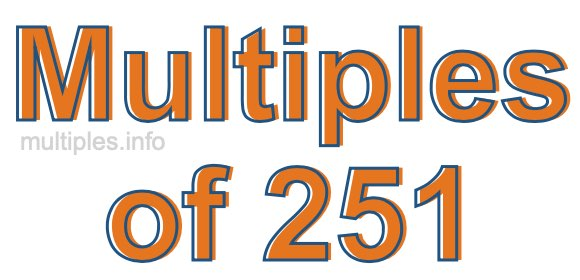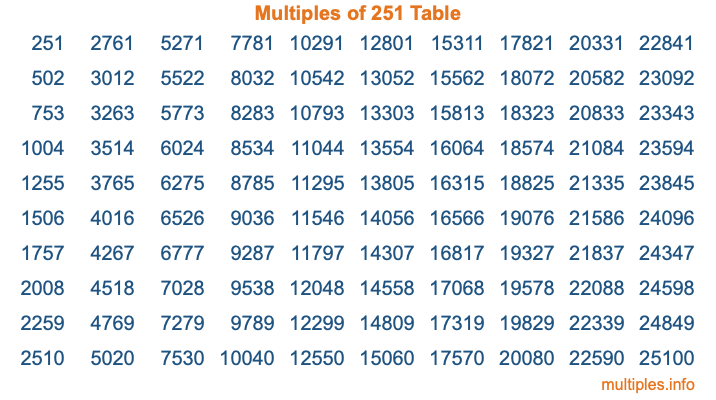Multiples of 251Welcome to the Multiples of 251 page. Here we will first teach you everything you will ever need to know about the multiples of 251, and then give you a study guide summary of everything we taught you to make sure you remember it all. Use this page to look up facts and learn information about the multiples of 251. This page will make you a multiples of two hundred fifty-one expert!

Definition of Multiples of 251
Multiples of 251 are all the numbers that when divided by 251 equal an integer. Each of the multiples of 251 are called a multiple. A multiple of 251 is created by multiplying 251 by an integer.

Therefore, to create a list of multiples of 251, you start with 1 multiplied by 251, then 2 multiplied by 251, then 3 multiplied by 251, and so on for as long as you want. Thus, the list of the first five multiples of 251 is 251, 502, 753, 1004, and 1255. To see a larger list of multiples of 251, see the printable image of Multiples of 251 further down on this page. We also have a category where you can choose any nth multiple of 251.

Multiples of 251 Checker
The Multiples of 251 Checker below checks to see if any number of your choice is a multiple of 251. In other words, it checks to see if there is any number (integer) that when multiplied by 251 will equal your number. To do that, we divide your number by 251. If the the quotient is an integer, then your number is a multiple of 251.

Is  a multiple of 251?

Least Common Multiple of 251 and ...
A Least Common Multiple (LCM) is the lowest multiple that two or more numbers have in common. This is also called the smallest common multiple or lowest common multiple and is useful to know when you are adding our subtracting fractions. Enter one or more numbers below (251 is already entered) to find the LCM.

Check out our LCM Calculator if you need more details about the Least Common Multiple or if you need the LCM for different numbers for adding and subtraction fractions.

nth Multiple of 251
As we stated above, 251 is the first multiple of 251, 502 is the second multiple of 251, 753 is the third multiple of 251, and so on. Enter a number below to find the nth multiple of 251.

th multiple of 251

Multiples of 251 vs Factors of 251
251 is a multiple of 251 and a factor of 251, but that is where the similarities end. All postive multiples of 251 are 251 or greater than 251. All positive factors of 251 are 251 or less than 251.

Below is the beginning list of multiples of 251 and the factors of 251 so you can compare:

Multiples of 251: 251, 502, 753, 1004, 1255, etc.

Factors of 251: 1, 251

As you can see, the multiples of 251 are all the numbers that you can divide by 251 to get a whole number. The factors of 251, on the other hand, are all the whole numbers that you can multiply by another whole number to get 251.

It's also interesting to note that if a number (x) is a factor of 251, then 251 will also be a multiple of that number (x).

Multiples of 251 vs Divisors of 251
The divisors of 251 are all the integers that 251 can be divided by evenly. Below is a list of the divisors of 251.

Divisors of 251: 1, 251

The interesting thing to note here is that if you take any multiple of 251 and divide it by a divisor of 251, you will see that the quotient is an integer.

Multiples of 251 Table
Below is an image of the first 100 multiples of 251 in a table. The table is in chronological order, column by column. The first column has the first ten multiples of 251, the second column has the next ten multiples of 251, and so on.The Multiples of 251 Table is also referred to as the 251 Times Table or Times Table of 251. You are welcome to print out our table for your studies.

Negative Multiples of 251
Although not often discussed or needed in math, it is worth mentioning that you can make a list of negative multiples of 251 by multiplying 251 by -1, then by -2, then by -3, and so on, to get the following list of negative multiples of 251:

-251, -502, -753, -1004, -1255, etc.

Multiples of 251 Summary
Below is a summary of important Multiples of 251 facts that we have discussed on this page. To retain the knowledge on this page, we recommend that you read through the summary and explain to yourself or a study partner why they hold true.

There are an infinite number of multiples of 251.

A multiple of 251 divided by 251 will equal a whole number.

251 divided by a factor of 251 equals a divisor of 251.

The nth multiple of 251 is n times 251.

The largest factor of 251 is equal to the first positive multiple of 251.

251 is a multiple of every factor of 251.

251 is a multiple of 251.

A multiple of 251 divided by a divisor of 251 equals an integer.

251 divided by a divisor of 251 equals a factor of 251.

Any integer times 251 will equal a multiple of 251.

Multiples of a Number
Here you can get the multiples of another number, all with the same attention to detail as we did for multiples of 251 on this page.

Multiples of
Multiples of 252
Did you find our page about multiples of two hundred fifty-one educational? Do you want more knowledge? Check out the multiples of the next number on our list!